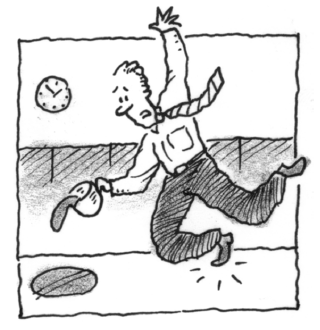### Home > APCALC > Chapter 7 > Lesson 7.1.4 > Problem7-37

7-37.

As clumsy Kenny pours his cold coffee on the floor, he notices that the growing puddle is circular. Let $A$ be the area of a circle with radius $r$ at time $t$. Write an equation that relates $\frac { d A } { d t }$ to $\frac { d r } { d t }$.

$A = πr^{2}$

Implicitly differentiate both sides of the area function WITH RESPECT TO TIME, $t$.